## Place (Field)

A place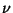of a number Field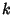is an Isomorphism class of field mapsonto a dense subfield of a nondiscrete locally compact Field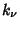.

In the function field case, letbe a function field of algebraic functions of one variable over a Field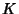. Then by a place in, we mean a subsetofwhich is the Ideal of nonunits of some Valuation Ring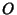over.

References

Chevalley, C. Introduction to the Theory of Algebraic Functions of One Variable. Providence, RI: Amer. Math. Soc., p. 2, 1951.

Knapp, A. W. Group Representations and Harmonic Analysis, Part II.'' Not. Amer. Math. Soc. 43, 537-549, 1996.